# tkinter的Canvas组件，绘画基本知识

Canvas组件，可以用来绘图，也可以用来制作动画，绘图，可以绘线条，圆，方形，多边形，制作动画，这是制作游戏的基础。

Canvas组件要学习的东西很多，参数也多，我觉得，我们可以先学一些基础的东西，等了解基础知识后，再深入学习。

Canvas组件，既然画线，画方框，画多边形，画圆，画文字，画组件等对象，你可以把Canvas组件当成容器组件来看待，先用“画”的方式来在画布里创建线，圆，框，文字，组件等，然后，再可经为这个组件设置各种属性：如更改坐标，改变颜色，当然你可以删除这些对象。

语法：Canvas（父组件，参数…….

```from tkinter import *

root=Tk()

can1=Canvas(root,width=200,height=150,background='lightblue')  # 浅蓝画布

can1.pack()

root.mainloop()```

create_line(x1, y1, x2, y2, …… xn,yn, 参数)

(X1,Y1)(x2,y2) ……. (xn,yn)  是不同点的坐标，画线的过程就是 (X1,Y1)，连接 (x2,y2)，再连接(x3,y3) …….再连接 (xn,yn)

arrow :

arrow=FIRST 起始点有箭头  arrow=LAST 最后一条线的末端有箭头

arrow=BOTH 2端都有箭头   默认是没有箭头

capstyle:

线条末端的样式：BUTT,  PROJECTING,  ROUND  默认为BUTT

dash:

画的线为虚线，虚线信息由元组来表现，元组的第1个数字为实线像素，第2个为空白像素，实线和空白交替使用元组的数字，用完后，再重新使用元组的第1个数字。如：

dash(5,1,1,1) 5像素的实线，1像素的空白，1像素的实线，1像素的空白

stipple: 绘制位图线条

widht : 线条宽度（粗细）

fill : 线条颜色

```from tkinter import *

root=Tk()

can1=Canvas(root,width=220,height=150,background='lightblue') # 浅蓝画布
can1.pack(fill=BOTH,expand=True)

can1.create_line(20,10,200,10) # 默认粗细线条
can1.create_line(20,35,200,35,width=5) # 粗线
can1.create_line(20,60,200,60,width=10,fill='red') # 红线
can1.create_line(20,85,200,85,dash=(5,2)) # 虚线
can1.create_line(20,110,200,110,arrow=BOTH) # 2端有箭头

root.mainloop()```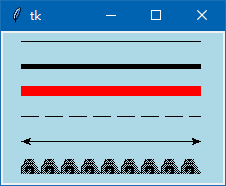create_rectangle(x1,y1, x2,y2, 参数)

(x1,y1) x2,y2）是矩形的左上角和右下角的坐标，由2个坐标决定矩形的大小。

dash: 矩形线条为虚线

fill :  矩形填充颜色

outline：矩形线条颜色

stipple：位图填充矩形

width: 矩形线条宽度

```from tkinter import *

root=Tk()

can1=Canvas(root,width=220,height=150,background='lightblue') # 浅蓝画布
can1.pack(fill=BOTH,expand=True)

can1.create_rectangle(30,10,90,60,fill='red',outline='green',width=4)  # 左上图

can1.create_rectangle(130,10,200,60,dash=(3,2)) # 右上图

fill='red', outline='blue',width=2) # 左下图

fill='blue', outline='red',width=2,dash=(3,2)) # 右下图

root.mainloop()```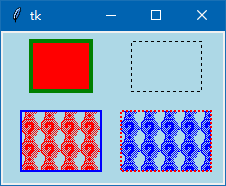create_arc( x1,y1, x2,y2, extent=angle, sytle=ARC, 参数 )

(x1,y1) x2,y2）决定一个矩形的左上角和右下角的坐标，而这个矩形决定圆弧的形状（弧度）。

extent: 跨度，即从圆弧的起始益到结束位置的角度，默认为90，范围是1359

style: 样式：扇形(‘pieslice’)  弓形(‘chord’) 弧形(‘arc’)  默认扇形

dash: 虚线

fill : 填充颜色

outline ：圆弧线条颜色

start : 圆弧起始位置，默认益是圆弧的右边开始，方向是逆时针

stipple : 位图圆弧

width : 圆弧线条宽度（粗细）

(x1,y1) x2,y2）坐标来决定的是画圆和圆弧还是画椭圆及椭圆弧

```from tkinter import *

root=Tk()

fr1=Frame(root,relief='ridge',borderwidth=1) # 不设置边线宽，无法显示
fr1.pack(fill=X)
fr2=Frame(root,relief='ridge',borderwidth=1) # 不设置边线宽，无法显示
fr2.pack()

var1=StringVar()
var1.set('弧形')

sca1=Scale(fr1,from_=0,to=359,orient=HORIZONTAL,label='起始偏移角度')

sca2=Scale(fr1,from_=0,to=359,orient=HORIZONTAL,label='圆心度(弧的跨度)')

def ok():
can1.delete('all')  # 清除所有对象
can1.create_rectangle(50,10,180,140,dash=(3,2)) # 画 正方形
can1.create_line(115,0,115,150,dash=(3,2),fill='yellow') # 画 水平中线
can1.create_line(40,75,190,75,dash=(3,2),fill='yellow') # 画 垂直中线

a=var1.get()
if a=='弓形':
b='chord'
elif a=='弧形':
b='arc'
elif a=='扇形':
b='pieslice'

can1.create_arc(50,10,180,140,style=b,start=sca1.get(),extent=sca2.get(),outline='red',width=4)
# 根据参数的不同，生成不同的圆，圆弧（弓形，扇形）

but1=Button(fr2,text=" 画：圆、弧线 ",command=ok)
but1.pack()

can1=Canvas(fr2,width=220,height=150,background='lightblue') # 浅蓝画布
can1.pack(fill=BOTH,expand=True)

ok()

root.mainloop()```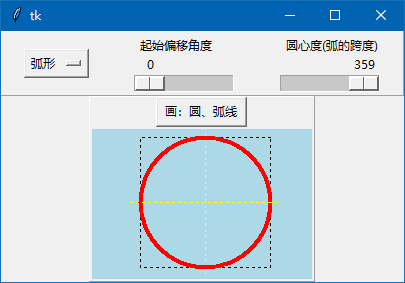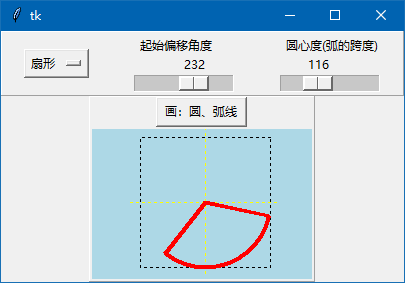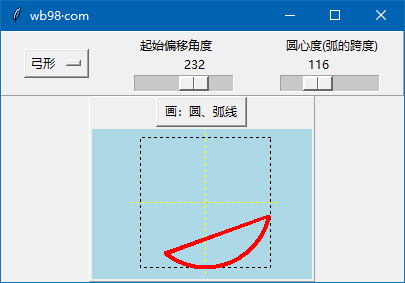create_oval( x1,y1, x2,y2, 参数 )

(x1,y1) x2,y2）坐标来决定的是画圆 还是画椭圆。

```
from tkinter import *

root=Tk()

can1=Canvas(root,width=270,height=150,background='lightblue') # 浅蓝画布
can1.pack()

can1.create_rectangle(20,10,150,140,dash=(3,2),outline='green') # 画 正方形
can1.create_oval(20,10,150,140,width=4,fill='lightyellow') # 画 圆，填充浅黄

can1.create_rectangle(160,10,250,140,dash=(3,2),outline='green') # 画 长方形
can1.create_oval(160,10,250,140,width=4,fill='lightgreen') # 画 椭圆，填充浅绿

root.mainloop()```

运行结果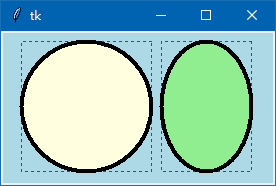create_polygon( x1,y1, x2,y2, …… xn,yn, 参数 )

(X1,Y1)(x2,y2) ……. (xn,yn)  是不同点的坐标。

```from tkinter import *

root=Tk()

can1=Canvas(root,width=460,height=200,background='lightblue') # 浅蓝画布
can1.pack(fill=BOTH,expand=True)

can1.create_polygon(10,10, 100,10, 50,80,fill='',outline='red') # 红框，透明填充
can1.create_line(10,100, 100,100, 50,180, fill='red') # 画线

can1.create_polygon(120,10, 180,30, 250,50, 200,80, 130,70, fill='lightyellow',outline='blue') # 蓝框，浅黄填充
can1.create_line(120,100, 180,120, 250,140, 200,170, 130,160 ,fill='blue') # 画线

can1.create_polygon(260,10, 370,30, 440,70, 380,90, 320,80, outline='green',width=5) # 绿框,默认为黑色填充
can1.create_line(260,110, 370,130, 440,170, 380,190, 320,180, fill='green',width=5) # 画线

root.mainloop()```

运行结果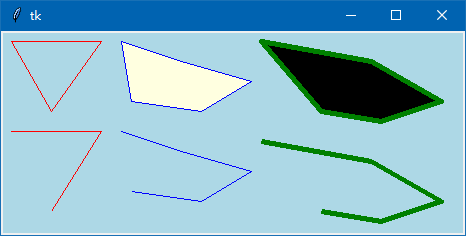fill=’’ 表示多边形填充的是透明色。如果没有写fill这个参数，默认是填充黑色。

create_text( x,y, text=字符文本, 参数 )

(x,y) 是字符文本在画布的坐标位置，但文本的起始位置要根据参数 anchor 来决定。

anchor 这个参数跟以前学的标签组件的anchor一样，有"n", "ne", "e", "se", "s", "sw", "w", "nw", "center" 9个选项，默认是center这个居中选项。

fill : 字符文本的颜色。

font : 字符文本的字体，大小。

justify : 多行显示时，靠那一边对齐。默认是LEFT

width : 这个参数类似于标签组件的wraplength参数，它决定在什么位置字符文本换行。

stipple : 指定一个位图用于填充，如果是空字符串，即字体为实心。

```。
from tkinter import *

root=Tk()

can1=Canvas(root,width=240,height=100,background='lightblue') # 浅蓝画布
can1.pack(fill=BOTH,expand=True)

can1.create_line(120,0, 120,100,fill='yellow') # 垂直中心线
can1.create_line(0,50, 240,50,fill='yellow') # 水平中心线

can1.create_text(120,50,text='中国在中间',font=('黑体',17,'bold'),fill='red') # anchor默认为center
can1.create_text(120,50,text='美国靠下边',font=('黑体',17,'bold'),fill='blue',anchor=NW)

root.mainloop()```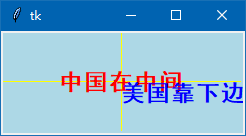“中国在中间”文本没有设置anchor参数，anchor默认为center，居中，这个文本的锚点是文本的正中间，正中间的坐标就是 x,y 这个坐标。

create_image( x,y, 参数 )

(x,y) 是插入图片的坐标位置，但图片的位置要根据参数 anchor 来决定，这点跟上面的说的插入文本是完全一样的。

create_window( x,y, 参数 )

(x,y) 坐标，anchor参数跟上面讲解知识完全一样。

window：这个参数又类似Text组件插入其他组件一样，插入的组件也一样可以响应事件。

```from tkinter import *

root=Tk()

can1=Canvas(root,width=240,height=100,background='lightblue') # 浅蓝画布
can1.pack(fill=BOTH,expand=True)

can1.create_line(120,0, 120,100,fill='yellow') # 垂直中心线
can1.create_line(0,50, 240,50,fill='yellow') # 水平中心线

but1=Button(root,text="按钮",command=lambda:print('被点击了')) # 先创建按钮

can1.create_window(120,50,window=but1) # 在正中间插入按钮
can1.create_window(120,50,window=ra1,anchor=NW) # 插入多选按钮

root.mainloop()```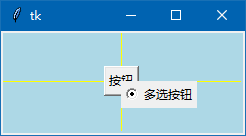```from tkinter import *
from tkinter.colorchooser import *

root=Tk() # 源码来自 wb98.com

fr1=Frame(root,relief='ridge',borderwidth=1) # 不设置边线宽，无法显示
fr1.pack(fill=X)

but1=Button(fr1,text="点击选择画笔颜色", relief='sunken', bg='red',

la1=Label(fr1,text='画笔粗细: ')
la1.pack(side=LEFT)

spin1=Spinbox(fr1,from_=1,to=20,width=6) # 画笔粗细
spin1.pack(side=LEFT)

but2=Button(fr1,text=" 清空画布 ",command=lambda:can1.delete('all')) # 清空画布

can1 = Canvas(root,width=400, height=200,background='white') # 白底画布
can1.pack(fill=BOTH,expand=True)

def paint(event): # 鼠标点击画点 或 按下鼠标移动画线
hb=int(spin1.get()) # hb是画笔的粗细
x1, y1 = (event.x - hb), (event.y - hb)  # 圆的左上角坐标
x2, y2 = (event.x + hb), (event.y + hb)  # 圆的右下角坐标
can1.create_oval(x1, y1, x2, y2,fill=but1['bg'], outline=but1['bg']) # 画圆 连线

can1.bind("<B1-Motion>", paint) # 按下鼠标移动画画
can1.bind("<Button-1>",paint) # 点击鼠标右键画点

root.mainloop()```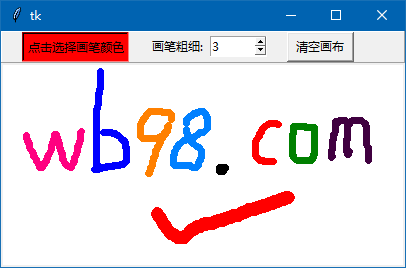tkinter中没有画点的方法，在上述代码中，是以画圆的方法来画点的，最小的粗细的画笔就是圆的左上角坐标跟右下角坐标是一样的。

Canvas组件还有一些参数没有说明，我自己也要慢慢学习，再来讲解。

<< 上一篇 下一篇 >>